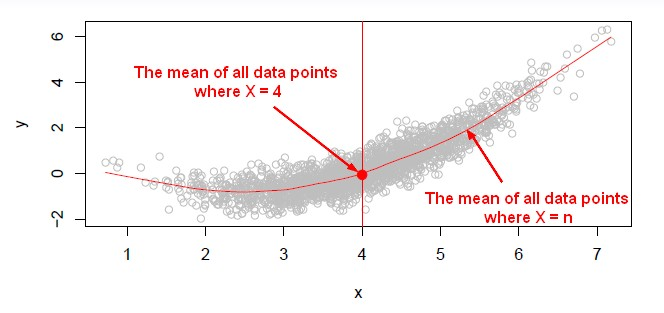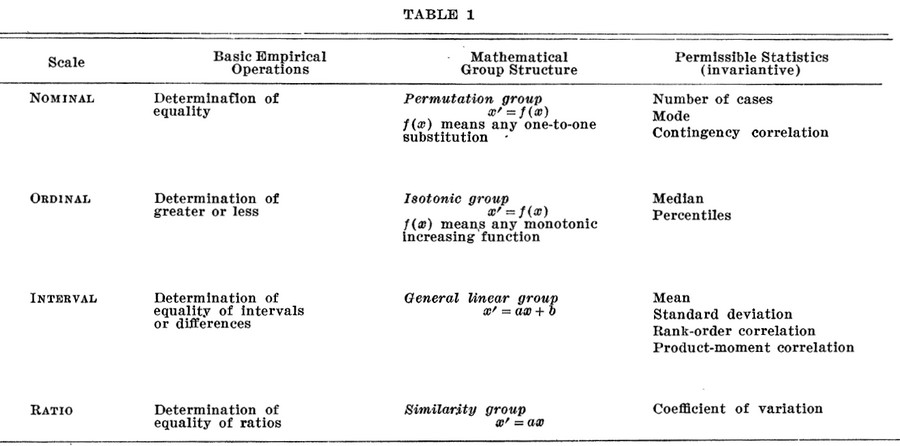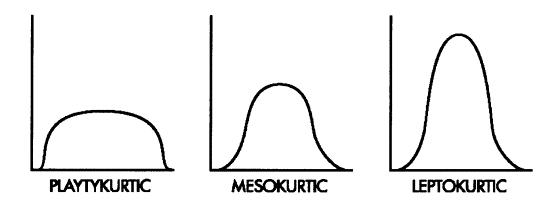# Statistics - Mode (Majority, Peak) (flatness, pointiness or modality)

The mode is a measure of center. It's the score that occurs most often.

Mode can be used for nominal variables (that's not true for Mean and Median).

Graphically, the peak of a histogram is the mode.

Mode:

Recommended PagesData Mining - Random forest

Random forest (or random forests) is a trademark term for an ensemble classifier that consists of many decision trees and outputs the class that is the mode of the classes output by individual trees. ...Distribution - Measures of (center|central tendency) (Mean, Median, Mode)

A Measure of central tendency is a measure that describes the middle or center point of a distribution. A good measure of central tendency is representative of the distribution. The mean, the median and...Function - (Aggregate | Aggregation)

Aggregate functions return a single value calculated or selected from values that are in a aggregation relationship (ie a set) This values are also known as summary because they try to summarize...Machine Learning - K-Nearest Neighbors (KNN) algorithm - Instance based learning

“Nearest‐neighbor” learning is also known as “Instance‐based” learning. K-Nearest Neighbors, or KNN, is a family of simple: classification and regression algorithms based on Similarity...Nominal Variable - Mode

SeeStatistics - (Data|Data Set) (Summary|Description) - Descriptive Statistics

Summary are a single value summarizing a array of data. They are: selected or calculated through reduction operations. They are an important element of descriptive analysis One of the most important...Statistics - (Scales of measurement|Type of variables)

According to Stevens theory (On the Theory of Scales of Measurement), the four scales of measurement are: nominal ordinal ...Statistics - Bimodal Distribution

A bimodal distribution has two modes.Statistics - Kurtosis (Distribution Tail extremity)

kurtosis is seen as measure of the “peakedness” of a distribution but it seems that it is not. Kurtosis as Peakedness, 1905 – 2014. R.I.P.,...